# Fit Advisor: Customizing Fit Formulas

For years, bicycle frame builders have used mathematical formulas to calculate appropriate bicycle geometry for a rider based on the rider's body measurements.

Some examples of how body measurements are taken by different builders are shown below.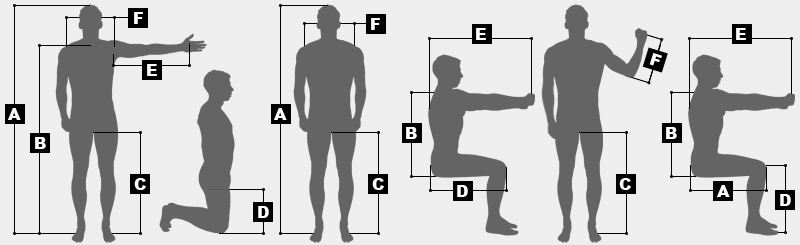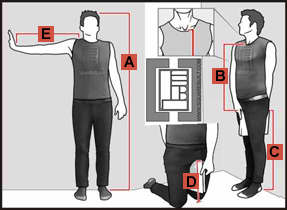For convenience, the three measurement diagrams above are included in BikeCAD Pro. However, BikeCAD Pro users can add their own images to the Fit Advisor as described here. An example of a customized measurement diagram is shown to the right.

If you would like to develop your own formulas for the Fit Advisor or modify the default formulas that are already there, start by selecting up to six body measurements to use as the basis for your fit scheme. In establishing these body measurements, be sure to reference anatomical body landmarks that will be repeatable for a variety of riders.

Next, find one or more riders that are already comfortable with their bike fits. By measuring these riders using our selected measurement scheme and then measuring the frame geometry of their current bikes, we'll be able to develop a fit scheme that would duplicate the fit of those riders when applied to other riders of different body dimensions.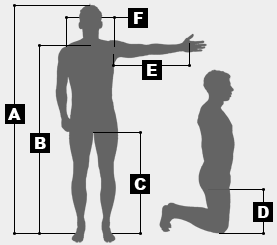As an example, let's assume that we're going to use the measuring scheme on the left.

Let's assume that we've already measured a rider with an inseam of 32.5" and that we've also measured their saddle height and found it to be 720mm. Because this rider is very comfortable with their saddle height, we'd like to determine the ratio between the rider's saddle height and their inseam length so that we can apply that ratio to the inseam length of other riders thereby determining an appropriate saddle height for them.

To determine the ratio, we must first convert the 32" inseam length to millimeters. This is done by multiplying 32.5" by 25.4. The equation would be:

32.5 * 25.4 = 825.5

The ratio of saddle height to inseam length would then be:

720 / 825.5 = 0.872

The ratio calculated above would be entered into the Fit Advisor customization screen as shown below: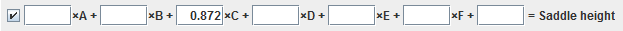The above formula calculates saddle height based on a single body dimension. Saddle height could alternatively be calculated from a combination of body dimensions. Dimension D (the femur length in this example) might also be factored in.

To demonstrate how more than one body dimension could be used to calculate a single bicycle dimension, consider a formula for rider compartment. Because rider compartment is a measure of the distance between the saddle and the handlebars, it is reasonable to assume that this dimension should be a function of a rider's torso length and arm length.

Let's assume that we are still developing formulas based on the measurement diagram above. The above diagram does not have a single measurement for torso. Instead, torso length could be calculated by subtracting dimension C from B.

Ultimately, if we'd like to calculate a value for rider compartment based on equal contributions from the rider's torso length and arm length. Our formula will need to be of the form:

x * B - x * C + x * E = rider compartment

Since x represents the ratio by which we'll be multiplying body dimensions B, C and E to obtain the recommended value for rider compartment, we can rearrange the equation to solve for x. The resulting equation would be:

x = rider compartment / (B - C + E)

Again, since we already have a benchmark rider with known body dimensions and we have measured the rider compartment on that rider's bike, we can substitute values for rider compartment, as well as B, C and E to solve the equation. Assuming our calculated value for x was 0.52 the resulting Fit Advisor formula would be entered as shown below: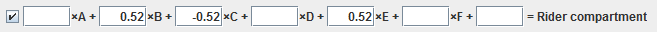Ideally, your final Fit Advisor formula would be obtained by averaging the calculated ratios from a number of benchmark riders and their respective bicycles.

Over time, you may feel the need to develop several more specific fit schemes. For example one fit scheme might cater to elite road racers, while another scheme might be more suitable for recreational road riders.

In developing BikeCAD Pro, the focus has been on creating a system where builders can apply their own fitting philosophies in an automated way. There is no expectation that builders will take the default values in the BikeCAD Pro Fit Advisor as gospel. Still, it is hoped that the default Fit Advisor values will yield good geometry for a wide range of riders. Therefore, if you have feedback or suggestions to improve the Fit Advisor in BikeCAD Pro it would be great if you could share your thoughts in the BikeCAD Forum.